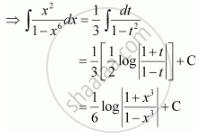Share

# Integrate the Functions X^2/(1 - X^6) - CBSE (Science) Class 12 - Mathematics

ConceptIntegrals of Some Particular Functions

#### Question

Integrate the functions x^2/(1 - x^6)

#### Solution

Let x3 = t

∴ 3x2 dx = dtIs there an error in this question or solution?

#### Video TutorialsVIEW ALL 

Solution Integrate the Functions X^2/(1 - X^6) Concept: Integrals of Some Particular Functions.
S